Do you like the article?
Share it with others —
Use new possibilities of MetaTrader 5

#### Similar articles# Custom Indicators in MQL5 for Newbies

MetaTrader 5Examples | 3 March 2010, 14:4037 83316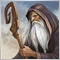### Introduction

The basis of deep understanding of any intellectual subject (no matter, mathematics, music or programming) is the study of its fundamentals. It's great when a similar study is started in a fairly young age, so the understanding of fundamentals is much easier, and knowledge is specific and comprehensive.

Unfortunately, the most of people begin to study financial and stock markets in a middle age, so the study isn't easy. In this article I'll try to help to overcome this initial barrier in understanding of MQL5 and in writing custom indicators for the MetaTrader 5 client terminal.

### SMA Indicator as a Simple Example

The most effective and rational way to study something is the solution of practical problems. As we are considering custom indicators, we will start with the study of the simple indicator that contains a code that illustrates the fundamentals of operation of indicator in MQL5.

As example, let's consider the most famous indicator of technical analysis - Simple Moving Average (SMA). Its calculation is simple:

SMA = SUM (CLOSE (i), MAPeriod) / MAPeriod

where:

• SUM — sum of values;
• CLOSE (i) — closing price of the i-th bar;
• MAPeriod — number of bars to average (averaging period).

Here is a code of this indicator free of any excesses:

```//+------------------------------------------------------------------+
//|                                                          SMA.mq5 |
//|                        Copyright 2009, MetaQuotes Software Corp. |
//|                                              http://www.mql5.com |
//+------------------------------------------------------------------+
#property indicator_chart_window
#property indicator_buffers 1
#property indicator_plots   1
#property indicator_type1   DRAW_LINE
#property indicator_color1  Red

input int MAPeriod = 13;
input int MAShift = 0;

double ExtLineBuffer[];
//+------------------------------------------------------------------+
//| Custom indicator initialization function                         |
//+------------------------------------------------------------------+
void OnInit()
{
SetIndexBuffer(0, ExtLineBuffer, INDICATOR_DATA);
PlotIndexSetInteger(0, PLOT_SHIFT, MAShift);
PlotIndexSetInteger(0, PLOT_DRAW_BEGIN, MAPeriod - 1);
}
//+------------------------------------------------------------------+
//| Custom indicator iteration function                              |
//+------------------------------------------------------------------+
int OnCalculate(const int rates_total, const int prev_calculated, const int begin, const double &price[])
{
if (rates_total < MAPeriod - 1)
return(0);

int first, bar, iii;
double Sum, SMA;

if (prev_calculated == 0)
first = MAPeriod - 1 + begin;
else first = prev_calculated - 1;

for(bar = first; bar < rates_total; bar++)
{
Sum = 0.0;
for(iii = 0; iii < MAPeriod; iii++)
Sum += price[bar - iii];

SMA = Sum / MAPeriod;

ExtLineBuffer[bar] = SMA;
}

return(rates_total);
}
//+------------------------------------------------------------------+```

And here is a result of its work in MetaTrader 5 client terminal: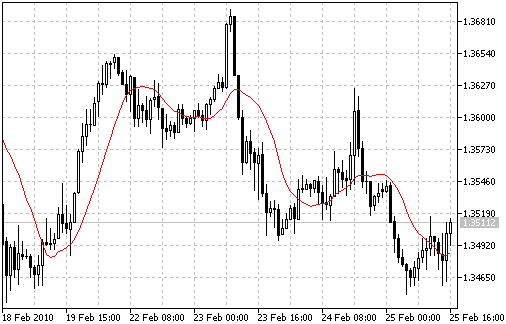First we need to consider two things - the purpose of each string of the code on the one hand, and interaction between this program code and the client terminal on the other hand.

At first glance at the indicator code, the eye catches objects like these:

```//+------------------------------------------------------------------+
//|                                                          SMA.mq5 |
//|                        Copyright 2009, MetaQuotes Software Corp. |
//|                                              http://www.mql5.com |
//+------------------------------------------------------------------+```
```//+------------------------------------------------------------------+
//| Custom indicator initialization function                         |
//+------------------------------------------------------------------+  ```
```//+------------------------------------------------------------------+
//| Custom indicator iteration function                              |
//+------------------------------------------------------------------+```
`//+------------------------------------------------------------------+`

It's necessary to note that they aren't related directly with the code, they are just comments, they are designed for code readability and shows a certain semantic content of some parts of this code. Of course, they could be removed from the code without any damage for its further simplification, but the code then would lose its laconic brevity in understanding. In our case, we deal with  single-line comments, which always start from a pair of characters "//" and end with a newline character.

It's clear that in comments the author can write everything necessary that will help to understand this code after some time. In our case, in the first part of the commented strings, there is a name of the indicator and information about its author, the second and third parts of comments are splitting the functions  OnInit() and OnCalculate(). The last line in the end simply closes the program code.

### Structure of SMA Code

So, as we see, the entire code of our indicator can be divided into 3 parts:

1. The code, that is written without brackets on the global level, it is located between the first two comments.
2. Description of the OnInit() function.

3. Description of the OnCalculate() function.

It's necessary to note that in programming the meaning of function is much wider than in mathematics. For example, in programming languages mathematical functions always receive some input parameters and return calculated values, in addition, functions in MQL5 also can perform some chart operations, trade operations, files operations, etc.

In fact, any indicator written in MQL5 always has some minimal user-written set of parts, the contents of which are individual and depend on the features of a created indicator.

Besides these components, the minimal set of functions can contain the description of another MQL5 function - OnDeInit():

```//+------------------------------------------------------------------+
//| Custom indicator deinitialization function                       |
//+------------------------------------------------------------------+
void OnDeinit(const int reason)
{

}```

In our case, it isn't necessary, so it's absent here.

### Interaction between SMA and the MetaTrader Client Terminal

Now let's consider the work of the compiled file SMA.ex5 that we obtained after pressing the "Compile" key in MetaEditor with opened SMA.mq5. It's necessary to note, that text files with extension .mq5 are just a source code in a text format, it should be compiled first to be used in the client terminal.

After attaching this indicator to a chart from the Navigator window, the MetaTrader will execute the code of the first part of the indicator. After that it will call the function OnInit() for a single execution of this function and further, at each new tick (after the new quote arrival) it will call the OnCalculate() function and consequently execute the code of this function.  IF OnDeInit() were present in the indicator, MetaTrader would call this function once after detaching the indicator from the chart or after the timeframe changing.

The meaning and purpose of all parts of the indicator are clear after this explanation. In the first part of the code on the global level there are some simple operators that are executed once after the start of the indicator. In addition there is a declaration of variables, that are "visible" in all blocks of the indicator and that remember their values while the indicator is on the chart.

The constants and functions that are executed once should be located inside the OnInit() function, because it would be inexpedient to place them in block of the function OnCalculate(). The code for the calculation of the indicator, that allows to calculate its values for each bar, should be placed in function OnCalculate().

The procedures that delete the useless garbage (if there is any) from the chart after the indicator is removed from it, should be placed inside of OnDeInit(). For example, it's necessary for the deletion of the graphic objects, created by the indicator.

After these explanations, we are ready to examine in details the code of the indicator, that has been considered above.

### Program Code of the SMA Indicator

The first group of code lines starts with the operator #property, that allows specifying additional parameters of indicator settings. The full list of possible program properties can be found in documentation of MQL5. If necessary, it's possible to write additional properties of the indicator. In our case we have 5 lines of the code, the purpose of each line is described in comments:

```//---- the indicator will be plotted in the main window
#property indicator_chart_window
//---- one buffer will be used for the calculations and plot of the indicator
#property indicator_buffers 1
//---- only one graphic plot is used
#property indicator_plots   1
//---- the indicator should be plotted as a line
#property indicator_type1   DRAW_LINE
//---- the color of the indicator's line is red
#property indicator_color1  Red ```

Note that there are no semicolons (";") at the ends of the lines. The reason is the following: in fact, in our case it's definition of constants, but presented in another way.

Our simple moving average has only 2 parameters, that can be changed by a user - it's an averaging period and horizontal shift (in bars) of the indicator along the time axes. These two parameters should be declared as input variables of the indicator, as it has been declared in two further code lines:

```//---- indicator input parameters
input int MAPeriod = 13; //averaging period
nput int MAShift = 0; //horizontal shift (in bars)
```

Note that after the declaration of these input parameters there are comments, and these comments will be visible as names of input parameters in the "Properties" window of the indicator: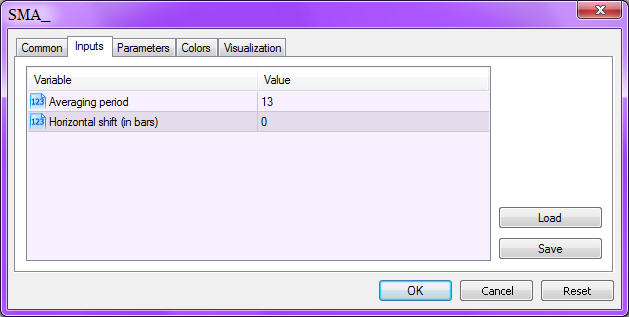In our case, these names are much clearer, than the variable names of the indicator. So, these comments should be simple.

And the last code line that hasn't any brackets is the declaration of the dynamic array ExtLineBuffer[].

```//---- the declaration of the dynamic array
//that will be used further as an indicator's buffer
double ExtLineBuffer[];
```

It has been declared as a global variable because of the several reasons.

First of all, this array should be converted into the indicator buffer, it's implemented in the block of the OnInit() function. Second, the indicator buffer itself will be used inside the OnCalculate() function. Third, this array will store the values of the indicator, that will be plotted as a curve on the chart. Because of the fact, that it has been declared as a global variable, it's available for all blocks of the indicator, and it stores its values all the time until the indicator is detached from the chart.

The content of the OnInit() function is presented by just 3 operators, they are built-in functions of the MetaTrader client terminal.

The call of the first function assigns the zeroth indicator buffer with one dimensional dynamic array ExtLineBuffer[]. Two calls of another function with different values of input parameters allow to shift the indicator along the price axis and allows to specify its plotting from the bar with number MAPeriod.

```void OnInit()
{
//----+
//---- assign the dynamic array ExtLineBuffer with 0th indicator's buffer
SetIndexBuffer(0,ExtLineBuffer,INDICATOR_DATA);
//---- set plot shift along the horizontal axis by MAShift bars
PlotIndexSetInteger(0,PLOT_SHIFT,MAShift);
//---- set plot begin from the bar with number MAPeriod
PlotIndexSetInteger(0,PLOT_DRAW_BEGIN,MAPeriod);
//----+
}```

The last call of the PlotIndexSetInteger() function passes the value equal to MAPeriod (via the parameter begin of the function OnCalculate()) to another indicator, if it is applied to the values of our indicator. The logic is simple, there is nothing to average in the first MaPeriod-1 bars, that's why the plotting of this indicator is useless. However, this value should be passed to shift the origin of the calculations of another indicator.

It isn't a full list of built-in functions, that are used in custom indicators and can be located in this block of the indicator. See MQL5 documentation for the details.

Finally, let's consider the code of the OnCalculate() function. There isn't any custom calls in this function like the function OnInit() because these functions are called by the MetaTrader client terminal. Because of this reason, the input parameters of the function are declared as constants.

```int OnCalculate(
const int rates_total,    // number of available bars in history at the current tick
const int prev_calculated,// number of bars, calculated at previous tick
const int begin,          // index of the first bar
const double &price[]     // price array for the calculation
)
```

These input parameters cannot be changed, their values are passed by the client terminal for the further use in the code of this function. The input variables of OnCalculate are described in documentation of MQL5. The function OnCalculate() returns its values for the client terminal using the return(rates_total) function. The client terminal receives this value of the current tick after the execution of OnCalculate() and passes the returned value to another parameter prev_calculated. So, it's always possible to determine the range of bar indexes and perform the calculations at once only for new values of the indicator, that have appeared after the previous tick.

It's necessary to note that the bars ordering in MetaTrader client terminal is performed from left to right, so the very old bar (the left), presented at chart has index 0, the next has index 1, etc. The elements of the buffer ExtLineBuffer[] have the same ordering.

The simple code structure inside the function OnCalculate of our indicator is universal and typical for many technical analysis indicators. So, let's consider it in details. The liogic of OnCalcualte() function is:

1. Check for the presence of bars, necessary for the calculations.
2. Declaration of local variables.
3. Get the index of starting bar for the calculation.
4. The main loop of the calculation of the indicator
5. Return the value of rates_total to the client terminal by using the operator return().

I think that the first term is clear. For example, if the averaging period of Moving Average is equal to 200, but the client terminal has only 100 bars, it isn't necessary to perform calculation because there are no bars, sufficient for the calculation. So we have to return 0 to the client terminal using the operator return.

```//---- check for the presence of bars, sufficient for the calculation
if(rates_total<MAPeriod-1+begin)
return(0);```

Our indicator can be applied to the data of some other indicator, that also can have some minimal number of bars for the calculation.  The use of the constant begin is necessary to take into account this fact. See the article Applying One Indicator to Another for the details.

The local variables, declared in this block are necessary only for the intermediate calculations inside the OnCalculate() function. These variables are released from the computer RAM after the call of the function.

```//---- declaration of local variables
int first,bar,iii;
double Sum,SMA;```

It's necessary to be careful with the start index of the main loop (variable first). At the first call of the function (we can determine it by value of parameter prev_calculated) we have to perform the calculation of indicator values for all the bars. For all further ticks of the client terminal we have to perform the calculation only for the new bars appeared. It is done by 3 code lines:

```//---- calculation of starting index first of the main loop
if(prev_calculated==0) // check for the first start of the indicator
first=MAPeriod-1+begin; // start index for all the bars
else first=prev_calculated-1; // start index for the new bars
```

The range of the variable changes in the main loop operator of indicator re-calculation has been already discussed.

```//---- main loop of the calculation
for(bar=first;bar<rates_total;bar++)```

The bar processing in the main loop is performed in increasing order (bar++), in other words, from left to right, as a natural and right way. In our indicator it could be implemented in another way (in reverse order). It's better to use the increasing order in indicators. The variable of the main loop is named as "bar", but many programmers prefer to use the name "i". I prefer to use the "bar", because it makes code clearer and readable.

The averaging algorithm, that has been implemented in the main loop, is simple.

```     {
Sum=0.0;
//---- summation loop for the current bar averaging
for(iii=0;iii<MAPeriod;iii++)
Sum+=price[bar-iii]; // Sum = Sum + price[bar - iii]; // eqaual to

//---- calculate averaged value
SMA=Sum/MAPeriod;

//---- set the element of the indicator buffer with the value of SMA we have calculated
ExtLineBuffer[bar]=SMA;
}```

In the second loop, we are performing the cumulative summation of the prices from the previous bars of the period and dividing it by this averaging period. As a result we have the final value of SMA.

After the main loop is finished, the OnCalculate function returns the number of available bars from the variable rates_total. In the next call of the OnCalculate() function, this value will be passed by the client terminal to the variable prev_calculated. This value, decreased by 1, will be used as a start index for the main loop.

Here is the full source code of the indicator with detailed comments for each code line:

```//+------------------------------------------------------------------+
//|                                                          SMA.mq5 |
//|                        Copyright 2009, MetaQuotes Software Corp. |
//|                                              http://www.mql5.com |
//+------------------------------------------------------------------+
//---- the indicator will be plotted in the main window
#property indicator_chart_window
//---- one buffer will be used for the calculations and plot of the indicator
#property indicator_buffers 1
//---- only one graphic plot is used
#property indicator_plots   1
//---- the indicator should be plotted as a line
#property indicator_type1   DRAW_LINE
//---- the color of the indicator's line is red
#property indicator_color1  Red

//---- indicator input parameters
input int MAPeriod = 13; //Averaging period
input int MAShift = 0; //Horizontal shift (in bars)

//---- the declaration of the dynamic array
//that will be used further as an indicator's buffer
double ExtLineBuffer[];
//+------------------------------------------------------------------+
//| Custom indicator initialization function                         |
//+------------------------------------------------------------------+
void OnInit()
{
//----+
//---- assign the dynamic array ExtLineBuffer with 0th indicator's buffer
SetIndexBuffer(0,ExtLineBuffer,INDICATOR_DATA);
//---- set plot shift along the horizontal axis by MAShift bars
PlotIndexSetInteger(0,PLOT_SHIFT,MAShift);
//---- set plot begin from the bar with number MAPeriod
PlotIndexSetInteger(0,PLOT_DRAW_BEGIN,MAPeriod);
//----+
}
//+------------------------------------------------------------------+
//| Custom indicator iteration function                              |
//+------------------------------------------------------------------+
int OnCalculate(
const int rates_total,    // number of available bars in history at the current tick
const int prev_calculated,// number of bars, calculated at previous tick
const int begin,          // index of the first bar
const double &price[]     // price array for the calculation
)
{
//----+
//---- check for the presence of bars, sufficient for the calculation
if (rates_total < MAPeriod - 1 + begin)
return(0);

//---- declaration of local variables
int first, bar, iii;
double Sum, SMA;

//---- calculation of starting index first of the main loop
if(prev_calculated==0) // check for the first start of the indicator
first=MAPeriod-1+begin; // start index for all the bars
else first=prev_calculated-1; // start index for the new bars

//---- main loop of the calculation
for(bar = first; bar < rates_total; bar++)
{
Sum=0.0;
//---- summation loop for the current bar averaging
for(iii=0;iii<MAPeriod;iii++)
Sum+=price[bar-iii]; // It's equal to: Sum = Sum + price[bar - iii];

//---- calculate averaged value
SMA=Sum/MAPeriod;

//---- set the element of the indicator buffer with the value of SMA we have calculated
ExtLineBuffer[bar]=SMA;
}
//----+
return(rates_total);
}
//+------------------------------------------------------------------+```

This form of code is much easier to understand and read.

I would like to outline another feature that can be used to simplify the understanding of code. You can use spaces and empty lines to make it clear.

### Conclusion

That's all about the interaction between the code of the custom indicator and the MetaTrader client terminal. Of course, the subject is much wider than what we have considered, the aim of this article is to help newbies to understand the fundamentals, so see documentation for the details.

Translated from Russian by MetaQuotes Ltd.
Original article: https://www.mql5.com/ru/articles/37

Attached files |
sma.mq5 (1.78 KB)
sma_.mq5 (3.32 KB)

#### Other articles by this author

Last comments | Go to discussion (16)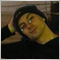| 2 Dec 2021 at 19:16

And one more. Why should I start calculating from the bar with the number (prev_calculated - 1), if I already calculated the indicator prev_calculated times?

For example, I calculated the indicator 5 times for the bars 0, 1, 2, 3 and 4. I don't need to calculate it on the bar 4 again. I should continue from the bar 5.| 3 Dec 2021 at 14:43
LRDPRDX #:

And one more. Why should I start calculating from the bar with the number (prev_calculated - 1), if I already calculated the indicator prev_calculated times?

For example, I calculated the indicator 5 times for the bars 0, 1, 2, 3 and 4. I don't need to calculate it on the bar 4 again. I should continue from the bar 5.

Ok. I think I am not correct here, because the 4th bar might have been changed since my last calculation. So the (prev_calculated - 1) looks right.| 23 Feb 2022 at 23:04
LRDPRDX #:

I don't understand. Suppose

rates_total == MA_Period - 1

and this is the first call of the function so

prev_calculated == 0

and also begin == 0

then the condition in the first if (rates_total < MA_Period - 1 + begin) evaluates to false

then

first = MA_Period - 1 would be executed

but then NO CALCULATIONS because the condition in the first for loop evaluates to false.

I think the first if condition should be

if( rates_total < MA_Period + begin )

am I missing something here?| 23 Feb 2022 at 23:07
I'm newbie here so I don't know how to this..and how to start..can you teach me how? And how this will work?| 5 Mar 2022 at 18:16
nellypingos #:
I'm newbie here so I don't know how to this..and how to start..can you teach me how? And how this will work?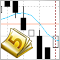How to call indicators in MQL5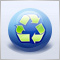The Order of Object Creation and Destruction in MQL5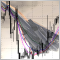Event handling in MQL5: Changing MA period on-the-fly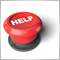MQL5.community - User Memo